Publicité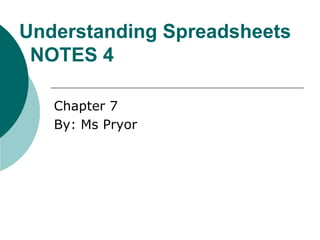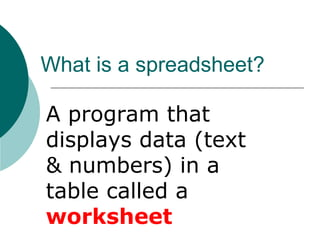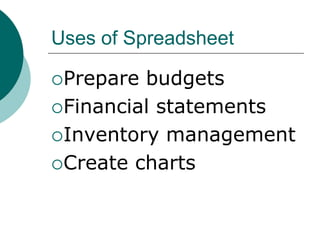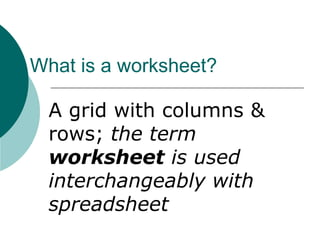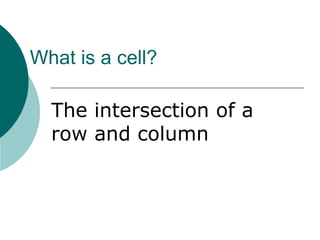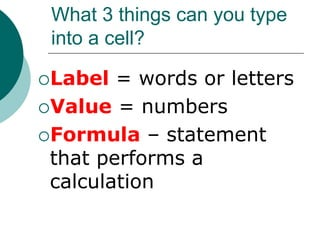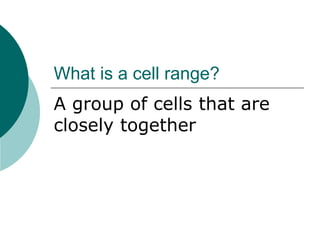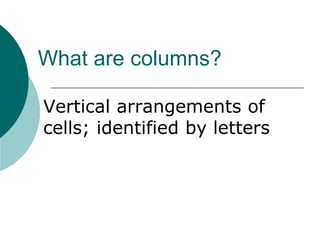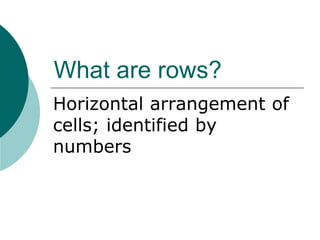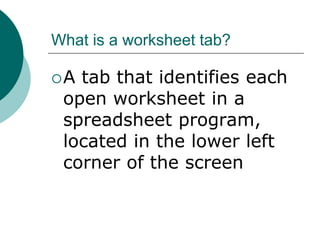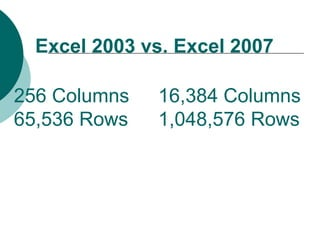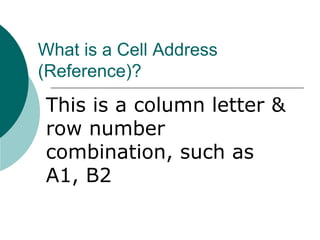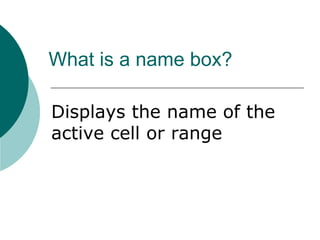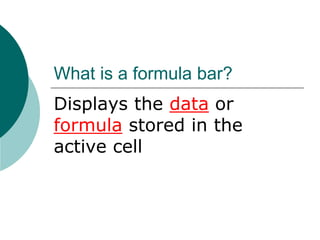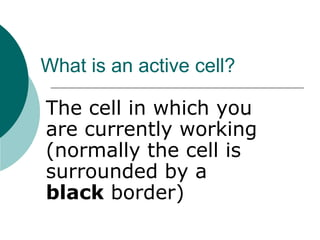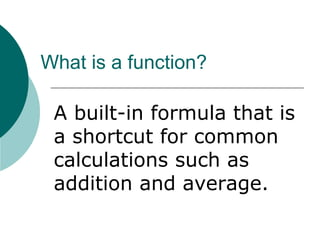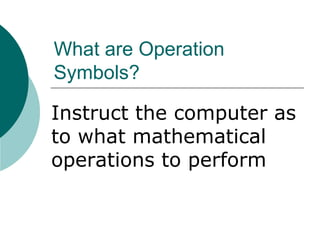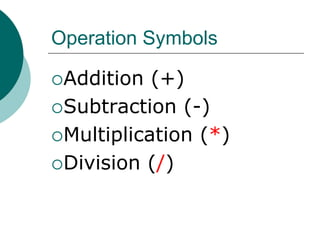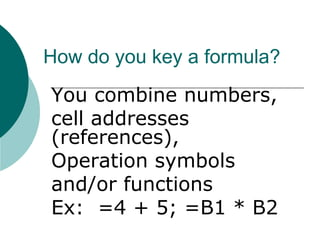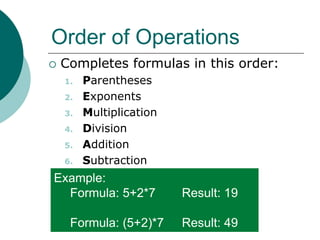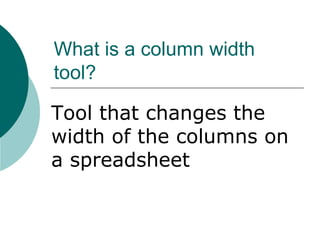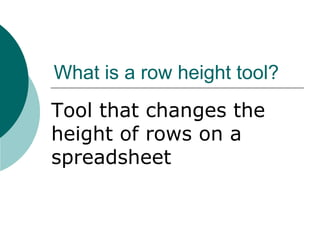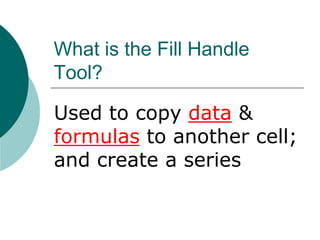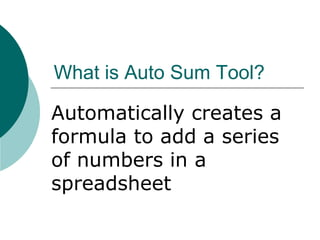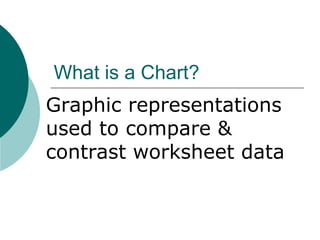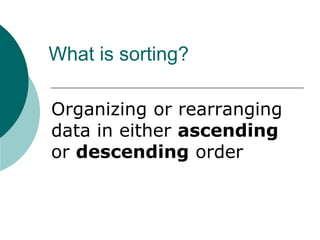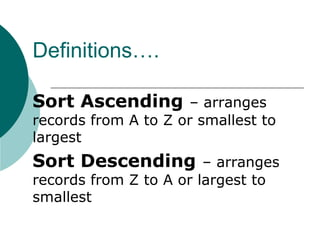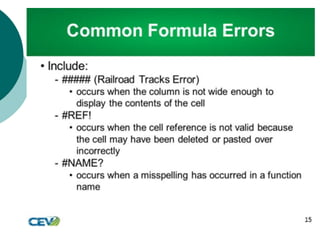1 sur 28
Publicité

### Lesson6 (1).ppt

1. Understanding Spreadsheets NOTES 4 Chapter 7 By: Ms Pryor
2. What is a spreadsheet? A program that displays data (text & numbers) in a table called a worksheet
3. Uses of Spreadsheet Prepare budgets Financial statements Inventory management Create charts
4. What is a worksheet? A grid with columns & rows; the term worksheet is used interchangeably with spreadsheet
5. What is a cell? The intersection of a row and column
6. What 3 things can you type into a cell? Label = words or letters Value = numbers Formula – statement that performs a calculation
7. What is a cell range? A group of cells that are closely together
8. What are columns? Vertical arrangements of cells; identified by letters
9. What are rows? Horizontal arrangement of cells; identified by numbers
10. What is a worksheet tab? A tab that identifies each open worksheet in a spreadsheet program, located in the lower left corner of the screen
11. Excel 2003 vs. Excel 2007 256 Columns 16,384 Columns 65,536 Rows 1,048,576 Rows
12. What is a Cell Address (Reference)? This is a column letter & row number combination, such as A1, B2
13. What is a name box? Displays the name of the active cell or range
14. What is a formula bar? Displays the data or formula stored in the active cell
15. What is an active cell? The cell in which you are currently working (normally the cell is surrounded by a black border)
16. What is a function? A built-in formula that is a shortcut for common calculations such as addition and average.
17. What are Operation Symbols? Instruct the computer as to what mathematical operations to perform
18. Operation Symbols Addition (+) Subtraction (-) Multiplication (*) Division (/)
19. How do you key a formula? You combine numbers, cell addresses (references), Operation symbols and/or functions Ex: =4 + 5; =B1 * B2
20. Order of Operations  Completes formulas in this order: 1. Parentheses 2. Exponents 3. Multiplication 4. Division 5. Addition 6. Subtraction Example: Formula: 5+2*7 Result: 19 Formula: (5+2)*7 Result: 49
21. What is a column width tool? Tool that changes the width of the columns on a spreadsheet
22. What is a row height tool? Tool that changes the height of rows on a spreadsheet
23. What is the Fill Handle Tool? Used to copy data & formulas to another cell; and create a series
24. What is Auto Sum Tool? Automatically creates a formula to add a series of numbers in a spreadsheet
25. What is a Chart? Graphic representations used to compare & contrast worksheet data
26. What is sorting? Organizing or rearranging data in either ascending or descending order
27. Definitions…. Sort Ascending – arranges records from A to Z or smallest to largest Sort Descending – arranges records from Z to A or largest to smallest
Publicité